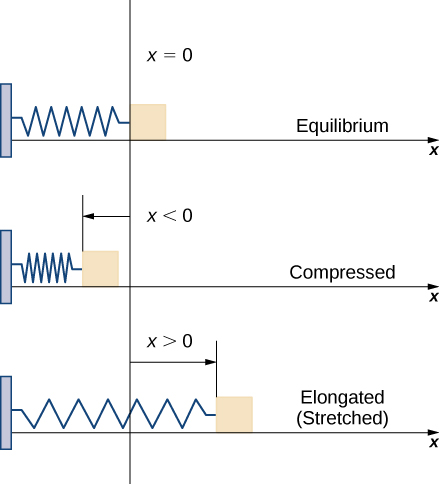# 6.5 Physical applications  (Page 3/11)

 Page 3 / 11
${W}_{i}\approx F\left({x}_{i}^{*}\right)\left({x}_{i}-{x}_{i-1}\right)=F\left({x}_{i}^{*}\right)\text{Δ}x.$

Therefore, the work done over the interval $\left[a,b\right]$ is approximately

$W=\sum _{i=1}^{n}{W}_{i}\approx \sum _{i=1}^{n}F\left({x}_{i}^{*}\right)\text{Δ}x.$

Taking the limit of this expression as $n\to \infty$ gives us the exact value for work:

$W=\underset{n\to \infty }{\text{lim}}\sum _{i=1}^{n}F\left({x}_{i}^{*}\right)\text{Δ}x={\int }_{a}^{b}F\left(x\right)dx.$

Thus, we can define work as follows.

## Definition

If a variable force $F\left(x\right)$ moves an object in a positive direction along the x -axis from point a to point b , then the work    done on the object is

$W={\int }_{a}^{b}F\left(x\right)dx.$

Note that if F is constant, the integral evaluates to $F·\left(b-a\right)=F·d,$ which is the formula we stated at the beginning of this section.

Now let’s look at the specific example of the work done to compress or elongate a spring. Consider a block attached to a horizontal spring. The block moves back and forth as the spring stretches and compresses. Although in the real world we would have to account for the force of friction between the block and the surface on which it is resting, we ignore friction here and assume the block is resting on a frictionless surface. When the spring is at its natural length (at rest), the system is said to be at equilibrium. In this state, the spring is neither elongated nor compressed, and in this equilibrium position the block does not move until some force is introduced. We orient the system such that $x=0$ corresponds to the equilibrium position (see the following figure).A block attached to a horizontal spring at equilibrium, compressed, and elongated.

According to Hooke’s law    , the force required to compress or stretch a spring from an equilibrium position is given by $F\left(x\right)=kx,$ for some constant $k.$ The value of $k$ depends on the physical characteristics of the spring. The constant $k$ is called the spring constant and is always positive. We can use this information to calculate the work done to compress or elongate a spring, as shown in the following example.

## The work required to stretch or compress a spring

Suppose it takes a force of $10$ N (in the negative direction) to compress a spring $0.2$ m from the equilibrium position. How much work is done to stretch the spring $0.5$ m from the equilibrium position?

First find the spring constant, $k.$ When $x=-0.2,$ we know $F\left(x\right)=-10,$ so

$\begin{array}{ccc}\hfill F\left(x\right)& =\hfill & kx\hfill \\ \hfill -10& =\hfill & k\left(-0.2\right)\hfill \\ \hfill k& =\hfill & 50\hfill \end{array}$

and $F\left(x\right)=50x.$ Then, to calculate work, we integrate the force function, obtaining

$W={\int }_{a}^{b}F\left(x\right)dx={\int }_{0}^{0.5}50x\phantom{\rule{0.2em}{0ex}}dx={25{x}^{2}|}_{0}^{0.5}=6.25.$

The work done to stretch the spring is $6.25$ J.

Suppose it takes a force of $8$ lb to stretch a spring $6$ in. from the equilibrium position. How much work is done to stretch the spring $1$ ft from the equilibrium position?

$8$ ft-lb

## Work done in pumping

Consider the work done to pump water (or some other liquid) out of a tank. Pumping problems are a little more complicated than spring problems because many of the calculations depend on the shape and size of the tank. In addition, instead of being concerned about the work done to move a single mass, we are looking at the work done to move a volume of water, and it takes more work to move the water from the bottom of the tank than it does to move the water from the top of the tank.

We examine the process in the context of a cylindrical tank, then look at a couple of examples using tanks of different shapes. Assume a cylindrical tank of radius $4$ m and height $10$ m is filled to a depth of 8 m. How much work does it take to pump all the water over the top edge of the tank?

Given that u = tan–¹(y/x), show that d²u/dx² + d²u/dy²=0
find the limiting value of 5n-3÷2n-7
Use the first principal to solve the following questions 5x-1
175000/9*100-100+164294/9*100-100*4
mode of (x+4) is equal to 10..graph it how?
66
ram
6
ram
6
Cajab
what is domain in calculus
nelson
integrals of 1/6-6x-5x²
derivative of (-x^3+1)%x^2
(-x^5+x^2)/100
(-5x^4+2x)/100
oh sorry it's (-x^3+1)÷x^2
Misha
-5x^4+2x
sorry I didn't understan A with that symbol
find the derivative of the following y=4^e5x y=Cos^2 y=x^inx , x>0 y= 1+x^2/1-x^2 y=Sin ^2 3x + Cos^2 3x please guys I need answer and solutions
differentiate y=(3x-2)^2(2x^2+5) and simplify the result
Ga
72x³-72x²+106x-60
okhiria
y= (2x^2+5)(3x+9)^2
lemmor
solve for dy/dx of y= 8x^3+5x^2-x+5
192x^2+50x-1
Daniel
are you sure? my answer is 24x^2+10x-1 but I'm not sure about my answer .. what do you think?
Ga
24x²+10x-1
Ga
yes
ok ok hehe thanks nice dp ekko hahaha
Ga
hahaha 😂❤️❤️❤️ welcome bro ❤️
Ga
y= (2x^2+5)(3x+9)^2
lemmor
can i join?
Fernando
yes of course
Jug
can anyone teach me integral calculus?
Jug
it's just the opposite of differential calculus
yhin
of coursr
okhiria
but i think, it's more complicated than calculus 1
Jug
Hello can someone help me with calculus one...
Jainaba
find the derivative of y= (2x+3)raise to 2 sorry I didn't know how to put the raise correctly
8x+12
Dhruv
8x+3
okhiria
d the derivative of y= e raised to power x
okhiria
rates of change and tangents to curves
how can find differential Calculus
Kyaw
derivative of ^5√1+x
can you help with this f(×)=square roots 3-4
oscar
using first principle, find the derivative of y=cosx^3
Approximate root 4 without a calculator
Approximate root 4.02 without using a calculator
Tinkeu
2.03
temigbe
unit of energy
Safiyo
< or = or >4.00000001
Fufa
2.01
Cajab
+-2
PRATICK
find the integral of tan
sec
PRATICK
sec
PRATICK
tan
dipeshByByByBy